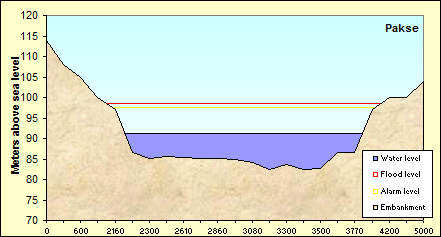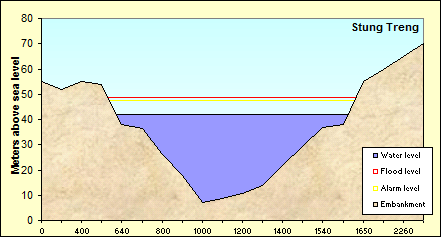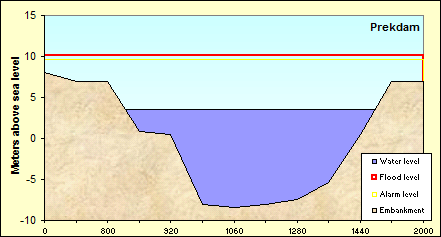## Monday, June 19, 2017

### Luang Prabang Water level on Monday, 19 June, 2017 at 07:00 AM = 6.57 m Forecast level on Tuesday, 20 June, 2017 = 6.69 m Flood level = 18.00 m Alarm level = 17.50 m All levels are above zero gauge (Zero gauge Luang Prabang = 267.195 m above MSL)Savannakhet Water level on Monday, 19 June, 2017 at 07:00 AM = 4.38 m Forecast level on Tuesday, 20 June, 2017 = 4.37 m Flood level = 13.00 m Alarm level = 12.00 m All levels are above zero gauge (Zero gauge Savannakhet = 125.022 m above MSL)Pakse Water level on Monday, 19 June, 2017 at 07:00 AM = 4.88 m Forecast level on Tuesday, 20 June, 2017 = 4.74 m Flood level = 12.00 m Alarm level = 11.00 m All levels are above zero gauge (Zero gauge Pakse = 86.49 m above MSL)StungTreng Water level on Monday, 19 June, 2017 at 07:00 AM = 5.09 m Forecast level on Tuesday, 20 June, 2017 = 5.02 m Flood level = 12.00 m Alarm level = 10.70 m All levels are above zero gauge (Zero gauge StungTreng = 36.79 m above MSL)Kratie Water level on Monday, 19 June, 2017 at 07:00 AM = 12.76 m Forecast level on Tuesday, 20 June, 2017 = 12.45 m Flood level = 23.00 m Alarm level = 22.00 m All levels are above zero gauge (Zero gauge Kratie = -1.08 m above MSL)Phnom Penh (Bassac) Water level on Monday, 19 June, 2017 at 07:00 AM = 4.25 m Forecast level on Tuesday, 20 June, 2017 = 4.14 m Flood level = 12.00 m Alarm level = 10.50 m All levels are above zero gauge (Zero gauge Phnom Penh (Bassac) = -1.02 m above MSL)Prek Kdam (Tonle Sap) Water level on Monday, 19 June, 2017 at 07:00 AM = 3.39 m Forecast level on Tuesday, 20 June, 2017 = 3.31 m Flood level = 10.00 m Alarm level = 9.50 m All levels are above zero gauge (Zero gauge Prek Kdam (Tonle Sap) = 0.08 m above MSL)Tan Chau Water level on Monday, 19 June, 2017 at 07:00 AM = 0.83 m Forecast level on Tuesday, 20 June, 2017 = 0.87 m Flood level = 4.50 m Alarm level = 3.50 m All levels are above zero gauge (Zero gauge Tan Chau = 0 m above MSL)Chau Doc (Bassac) Water level on Monday, 19 June, 2017 at 07:00 AM = 0.61 m Forecast level on Tuesday, 20 June, 2017 = 0.70 m Flood level = 4.00 m Alarm level = 3.00 m All levels are above zero gauge (Zero gauge Chau Doc (Bassac) = 0 m above MSL)****MAP OF LOWER MEKONG REGION - Source: MRC